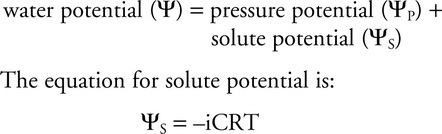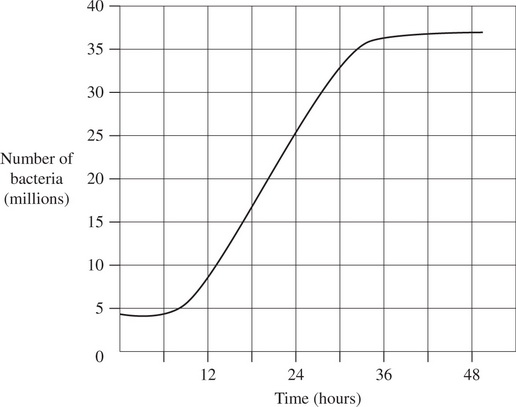# AP Biology Grid-Ins Practice Test 5

### Test Information6 questions9 minutes

1. In a certain species of plant, the allele to produce green melons (G) is dominant over the allele to produce yellow melons (g). A student performed a cross between a plant that produced green melons and a plant that produced yellow melons. When the student observed the next generation, the 94 seeds that were produced from the cross matured into 53 plants with green melons and 41 plants with yellow melons. Calculate the chi-squared value for the null hypothesis that the green-melon parent was heterozygous for the melon-color gene.

2. In a population of grasshoppers, the allele for tan color is dominant to the allele for green color. A drastic increase in rainfall leads to selection against the tan phenotype. When the rainy season ends, 23 percent of the remaining grasshoppers have the green phenotype. If this population is now in Hardy-Weinberg equilibrium, what will the frequency of the tan allele be in the next generation?

3. The bacteria that cause pimples can be grown in the lab using a suitable nutrient broth, where they will eventually achieve exponential growth. Using the graph that follows, calculate the mean rate of growth, in millions of bacteria per hour, during their exponential phase.

4. What is the water potential for a solution that is 0.1 M? The solution is in an open container. The equation for water potential is:where:

i = ionization constant (assume that it is 1)

C = molar concentration

R = pressure constant (R = 0.00831 liter MPa/mole K)

T = temperature in Kelvin (assume a room temperature of 293 K)

5. Determine the surface area-to-volume ratio for a cube that has a side length of 2.5 cm. The volume of a cube = (l)(w)(h). The surface area = 6 × area of a single side.

6. If CiVi = CfVf, where i is the initial solution concentration and f is the final concentration, how many milliliters of a 0.5 M glucose solution would you need in order to make 250 milliliters of a 0.1 M glucose solution?